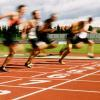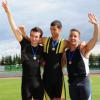#### You may also like### Decathlon: the Art of Scoring Points

How do decisions about scoring affect who wins a combined event such as the decathlon?### Secondary Sport Collection

This is our secondary collection of favourite mathematics and sport materials.

# 10 Olympic Starters

##### Age 16 to 18Challenge Level

Many of these problems involve an object moving under the influence of gravity and friction forces (such as air resistance). A first step in modelling is usually to neglect friction. In this case, the acceleration downwards due to gravity is about $9.8\mathrm{ms}^{-1}$. This leads to the simplifying modelling assumption that the downwards motion of a projectile is the reverse of the upwards motion. This can be used to calculate, for example, the speed of lift off by working out how fast an object will be landing when it hits the ground falling from a specified height (such as a pole-vault bar).

One of our summer students came up with these numbers:

1) Ignoring air resistance, if a long jumper could launch themselves at $45^{\circ}$ at a speed of $10.0\mathrm{ms}^{-1}$, they'd travel $\frac{10^2}{g}\mathrm{m}$ = $10.2\mathrm{m}$. (See here for a derivation). In reality, long jumpers would probably struggle to convert enough of their horizontal speed into the required vertical speed to travel on this trajectory. There's an interesting article here discussing how good a long jumper Usain Bolt could be.

2) Assume the gun is fired from exactly the same height as the target (though any height difference could be incorporated fairly simply into the model), and the bullet travels at a constant speed of $v= 400\mathrm{ms}^{-1}$. Suppose the bullet is fired at an angle $\alpha$ to the horizontal. The coordinates of the bullet are $$\left(v\cos(\alpha)t, v\sin(\alpha)t - \frac{g}{2}t^2\right)\;.$$ At time $t=T$ say, the bullet hits the target. If L is the distance to the target from the shooter, we find $\sin(2\alpha) = \frac{Lg}{v^2}$ (using $\sin(\alpha)=2\cos(\alpha)\sin(\alpha)$.) This gives the following values of $\alpha$ for the different distances:

 L (m) $\mathbf{\alpha (^{\circ})}$ 10 0.035 25 0.088 50 0.18

These seem to be very small numbers. However, if we included air resistance, the bullet would slow down during the motion and consequently hit the target lower than predicted here, so in reality there might need to be a noticably different aim for the different distances.

3) Suppose the athlete is only affected by the wind on the back and home straights, which are around $85\mathrm{m}$ in length, and they run exactly $1\mathrm{ms}^{-1}$ faster with the wind and $1\mathrm{ms}^{-1}$ slower when running against the wind. If the athelte's time in still conditions is $50\mathrm{s}$, then their average speed during the race is $8\mathrm{ms}^{-1}$. Their wind affected time using these assumptions would be:

$$\frac{(400 - 2\times85)\mathrm{m}}{8\mathrm{ms}^{-1}} + \frac{85}{8+1}\mathrm{s} + \frac{85}{8-1}\mathrm{s} = 50.3\mathrm{s}$$

i.e. their time is predicted to be $0.3\mathrm{s}$ slower.

We could incorporate other factors into the model. Air resistance, for example, is proportional to the speed squared, so could affect the time significantly. The wind would also affect the athlete around the bends of the track; here the wind strength would vary continuously. We've assumed the wind would affect the speeds along the straights symmetrically, in general this is likely to not be the case, as the athelete's performance may be reduced more than expected running into the wind.

4) A launch speed of $13\mathrm{ms}^{-1}$ seems a reasonable approximation. Neglecting air resistance, a throw with the velocity angled at $45^{\circ}$ to the ground would travel $\frac{13^2}{9.8}\mathrm{m} = 17.2\mathrm{m}$. The speed at which a shot putter can launch the shot depends on the launch angle. For more information, see here

5) Suppose the athlete has just equalled the world record of $6.14\mathrm{m}$. Assume the athlete has zero vertical speed at the top of the jump. Their speed at the bottom of the descent is therefore $\sqrt{0^2+2\times9.8\times6.14}\mathrm{ms}^{-1} = 11.0\mathrm{ms}^{-1}.$

6) A footballer can kick the ball at a speed of around $75\mathrm{mph}$. Assuming the football remains at this speed, it'll take $11\mathrm{m}\times\frac{1}{75\times\frac{1600}{3600}\mathrm{ms}^{-1}} = 0.33\mathrm{s}$ to reach the goal. The goalkeeper needs to have moved into position and be ready to save it in this time!

7) Assume for the time being, the vertical velocity and trajectory result in the ball landing in the centre of the hoop looking from above. In the horizontal plane, the athlete can shoot at an angle $\alpha$ off the centre, where $\alpha = \tan^{-1}\left(\frac{0.23}{4.6}\right) = 2.8^{\circ}$. Can you work out any restrictions on the angle to the horizontal of the initial velocity?

8) Using $s=ut+\frac{1}{2}at^2$ shows that the time (for a point particle) to fall from $10\mathrm{m}$ is $\sqrt{2\times 10/9.8}\mathrm{s} = 1.43\mathrm{s}$. Thus, the time taken to travel to a height of $10\mathrm{m}$ and back is $2\times1.43\mathrm{s} = 2.86\mathrm{s}$. If they need to rotate $720^{\circ}$ in that time, they need to rotate at $252^{\circ}\mathrm{s}^{-1}$.

9) If we assume all his mass is $0.9\mathrm{m}$ from the bar, then conservation of energy gives: $mg(2\times0.9) = \frac{1}{2}mv^2$, so $v \approx 6\mathrm{ms}^{-1}$.

10) A 'singles' tennis court measures $8.3\mathrm{m}$ by $23.8\mathrm{m}$. Suppose the player can serve at $50\mathrm{ms}^{-1}$. The straight serve takes $\frac{23.8}{50}\mathrm{s} = 0.476\mathrm{s}$ to get to the baseline. The diagonal serve travels $\sqrt{4.15^2 + 23.8^2}\mathrm{m} = 24.2\mathrm{m}$, so takes $0.49\mathrm{s}$.